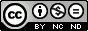### MATHEMATICAL MODEL MANIPULATOR ROBOTS

O. N. Krakhmalev

#### Аннотация

A mathematical model to describe the dynamics of manipulator robots. Mathematical model are the implementation of the method based on the Lagrange equation and using the transformation matrices of elastic coordinates. Mathematical model make it possible to determine the elastic deviations of manipulator robots from programmed motion trajectories caused by elastic deformations in hinges, which are taken into account in directions of change of the corresponding generalized coordinates. Mathematical model is approximated and makes it possible to determine small elastic quasi-static deviations and elastic vibrations. The results of modeling the dynamics by model are compared to the example of a two-link manipulator system. The considered model can be used when performing investigations of the mathematical accuracy of the manipulator robots.

#### Ключевые слова

manipulator robots; mathematical model; dynamic; elastic vibrations

#### Литература

Pavlov, B.I. and Ochirov, V.D., Vychislitel’nyi eksperiment v dinamike mashin i mekhanizmov [Computation Experiment in Machines and Mechanisms Dynamics]. Moscow: Nauka, 1981.

Kolovskii, M.Z. and Sloushch, A.V., Osnovy dinamiki promyshlennykh robotov [Foundations of Industrial Robots Dynamics]. Moscow: Nauka, 1988.

Chernousko F.L., Bolotnik N.N., Gradetsky V.G. Manipulation Robots. Dynamics, Control, and Optimization. Boca Raton: CRC Press, 1994.

Korendyasev, A.I., Salamandra, B.L., and Tyves, L.I., Teoreticheskie osnovy robototekhniki [Theoretical Foundations of Robotics]. Moscow: Nauka, 2006.

Krakhmalev, O.N., Matematicheskoe modelirovanie dinamiki manipulyatsionnykh sistem promyshlennykh robotov i kranov-manipulyatorov: monografiya [Mathematical Simulation of Manipulation System Dynamics for Industrial Robots and Articulated Cranes]. Bryansk: Bryansk. Gos. Tekh. Univ., 2012.

### Ссылки

• На текущий момент ссылки отсутствуют.

(c) 2016 International Journal of Advanced StudiesКонтент доступен под лицензией Creative Commons Attribution-NonCommercial-NoDerivs 4.0.

ISSN 2328-1391 (print), ISSN 2227-930X (online)